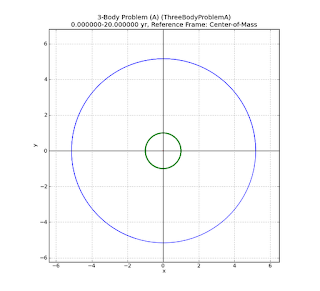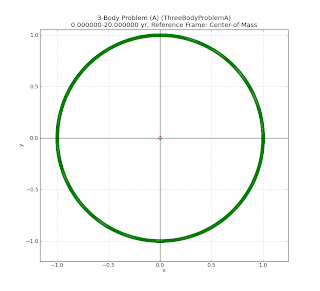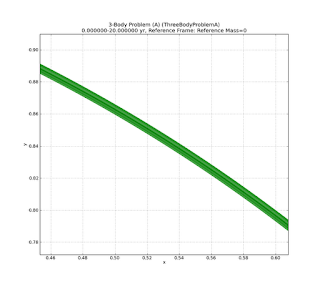## Sunday, March 4, 2012

### The Geocentrists' 3-Body "Problem"

Here I'll examine a 3-Body case of two large planets (which I'll call "Earth" & "Jupiter") orbiting a star ("Sun").

Technically, Kepler's laws no longer apply in their absolute form, because we have more than two bodies.  However, science always works with approximations, in part because there is no absolute perfect system of measurement.  We cannot measure to arbitrary high precision.  In these cases, what becomes important is does the technique work good enough to use for a given problem.  We don't know the exact locations of the electrons in the computer you're using to read this, but we know their position, and what they are doing, good enough to make the device work with reasonable reliability.

In this 3-body case,  the important question is not "do Kepler's laws apply", but by how much does the system deviate from Kepler's laws?

For this run of my N-body code (see Doin' Real Science: Simulating Particles), I'll choose a time step of 0.0001 years.

Time step = 0.0001 years = 0.036525 days ~ 52m 35s.

So my timestep size updates the simulation approximately each hour, and if I run the simulation for 200,000 steps, we have the equivalent of 20 years of total simulation time.

I'll also add an additional velocity of 0.01 AU/year in the y-direction to make the center-of-mass motion more evident.

Since in the real case, the deviations we're looking for are very small, we'll make our additional objects in the system much larger so the deviations will be more readily visible.  We'll choose the mass of the Sun for the primary body,  but we'll assign our "Earth" as 100 Earth masses (about 0.0003 solar masses), and our "Jupiter" as 10000 Earth masses (about 0.003 solar masses).  Here's the table of my input parameters:

Model:        3-Body Problem (A)
Particle Count=    3
Interaction=
Q    Object         Mass         ------------------ position -------------------
-------------------- velocity ---------------------
1)   0 Sun         1.00000e+00 Msun  x=( 0.000000e+00, 0.000000e+00, 0.000000e+00) AU
v=( 0.000000e+00, 1.000000e-02, 0.000000e+00) AU/yr
2)   0 Earth       3.00349e-04 Msun  x=( 1.000003e+00, 0.000000e+00, 0.000000e+00) AU
v=( 0.000000e+00, 6.293177e+00, 0.000000e+00) AU/yr
3)   0 Jupiter     3.00349e-03 Msun  x=( 5.202887e+00, 0.000000e+00, 0.000000e+00) AU
v=( 0.000000e+00, 2.764594e+00, 0.000000e+00) AU/yr

The '0' in the column labelled 'Q' means I've made the Sun and planets without a significant electric charge.

My python version runs this simulation in a few minutes on a 2.8GHz Intel.  Here's a plot of the resulting paths in the coordinate system of the input position and velocity values.(Click to enlarge)
The blue path is the orbit of "Jupiter" and the green path is the orbit of "Earth".  In the center, barely visible on this scale, is the red path representing the motion of the Sun.  We zoom in on this to better plot the motion:(Click to enlarge)
Here we see the red curve of the Sun's motion.  The dashed line is the motion of the center-of-mass of the system in the originally defined coordinate system.  The large wave motion of the red line is due to the balance with the motion of "Jupiter" around the center-of-mass.  However, you can see a smaller wiggle motion along the Sun curve.  This is due to the additional perturbation created by the motion of the "Earth" around the center-of-mass.

It's difficult to get a clear understanding in the input coordinate system, so let's convert all of our positions into the center-of-mass (CM) frame for the entire system, using the technique outlined in the earlier post (see Geocentrism & the Barycenter. II.):(Click to enlarge)
This looks a lot more interesting.  In the CM frame, the orbits now look much more like circles to the limit of the plotting precision.  The units in the x- and y-directions are AUs.
Let's take a closer look at the Earth's orbit (green):(Click to enlarge)
The orbit looks rather thick.  This is due to the Sun's motion around the CM and the Earth's orbit adjusting.  Remember that the Sun's motion around the CM is dominated by the motion of "Jupiter".  In the CM frame, the trajectory of the "Earth" wobbles around the Sun.  The forces to make this happen just work.

We can zoom in to the red curve in the center to see the motion of the Sun around the center-of-mass.(Click to enlarge)

The main circular motion of the CM is due to the massive planet, "Jupiter", but smaller wiggles are due to the Earth's motion.  The reaction force of the "Earth" on the Sun moves the Sun by a tiny amount, which is mathematically constrained to balance around the center of mass.  It is the superposition of these motions around the center-of-mass that allows us to detect multiple extrasolar planets by the radial velocity method (wikipedia).

Now we zoom back out to full scale again, and switch to a coordinate system so the Sun is fixed at the origin (0,0,0) of the coordinate system.(Click to enlarge)

At this scale, to the eye, the orbits of the "Earth" and "Jupiter" appear very circular.  I had chosen input positions and velocities consistent with circular orbits using Kepler's laws.  So far, a Keplerian approximation used for originally defining the orbits looks like it's holding up pretty good, at least to the pixel resolution of this plot.

We can take a closer look a the Earth's orbit in this Sun-centered coordinate system.(Click to enlarge)
Now the Sun is the red dot fixed at the center of the plot.  The orbit of "Earth" still looks pretty close to a circular orbit, extending to 1AU along along the coordinate axes, to the pixel resolution.  The shape in the center is the wiggling trajectory of the center-of-mass motion around the Sun in this frame.  Here we take a closer look:(Click to enlarge)
This motion mirrors the motion of the Sun around the around the center-of-mass (note previous figure).  By the way, on this scale, the radius of the Sun is about 695,500km/149,598,000km = 0.00465 AU so the center-of-mass lies far outside the surface of the Sun.

If we want to see some differences, we need to zoom in even more on the green curve of the Earth's orbit.(Click to enlarge)
At this scale, we see that the orbit path looks much thicker, like there might be overlapping paths.  So we zoom in some more:(Click to enlarge)
At this scale, each mark in the grid is 0.002AU = 2*149000 km = 299,000 km, less than the distance of the Earth from the Moon.  The difference in position for each orbit of the Earth is about 1/10 of this, or about 30,000 km.  Because I increased the planet masses, this deviation is actually far larger than it would be in the real case.

For this scale, this is actually pretty good accuracy for the technique utilized.  At full scale, we cannot easily distinguish the deviation of the orbit from a circle.  We must be very close to see it.  Part of the motion is due to the perturbations created by the presence of "Jupiter", but part is also due to the fact that each step in the calculation, the next position is computed only to a finite level of precision and some accuracy is lost.  Yet in spite of these errors, orbit is almost indistinguishable from the original defined orbit.

The notion that a theory or model must provide perfect precision is a delusion propagated by pseudo-scientists.  Models are always limited by our measuring precision, on both the input and output parameters.

Imagine driving down the road, at 65 mph, and seeing a sign for you destination, 130 miles away.  With this information, I estimate 2 hours to my destination.  Is that perfectly accurate?  No.  It does not include small changes in speed due to uphill or downhill travel, or even that tap on the brakes as you see the state trooper around the bend and realize you're travelling a bit over the speed limit.  Is my estimate of 2 hours inaccurate?  Yes.

But is my estimate of 2 hours useless?  Not really.  It is still perfectly acceptable for planning my travel, and like most data, can be revised as I get closer to my destination (and incorporate bathroom or gas stops, etc.)

The inaccuracy of the estimate does not invalidate the equation (time) = (distance travelled)/(speed).   This in itself is an approximation based on the assumption of constant speed.  But even if you are not travelling at constant speed, it does not become grossly wrong.

So it is with Kepler's Laws.  When applied to the other planets in the solar system, it is not perfectly exact - but it is not grossly wrong either.  The other bodies in the solar system must be much more massive to perturb the other planets sufficiently that Kepler's Laws become totally useless, contrary to Mr. Martin's pedantic claims.

"Pedantry and mastery are opposite attitudes toward rules. To apply a rule to the letter, rigidly, unquestioningly, in cases where it fits and in cases where it does not fit, is pedantry ... To apply a rule with natural ease, with judgment, noticing the cases where it fits, and without ever letting the words of the rule obscure the purpose of the action or the opportunities of the situation, is mastery." -George Polya, mathematician (1887-1985)

#### 1 comment:

W.T."Tom" Bridgman said...

365 Days of Astronomy has published a podcast on n-body problems.